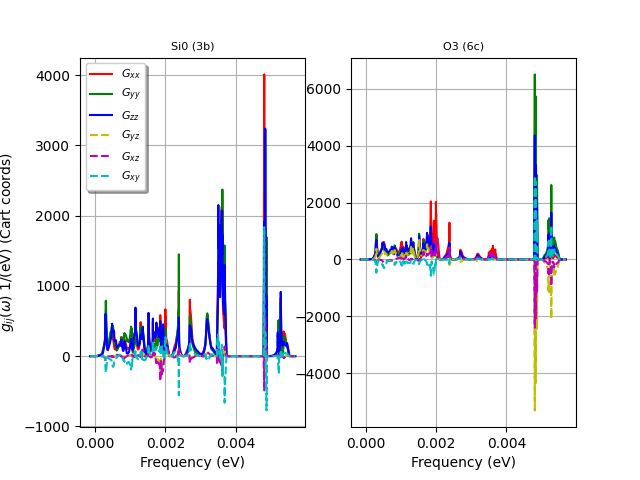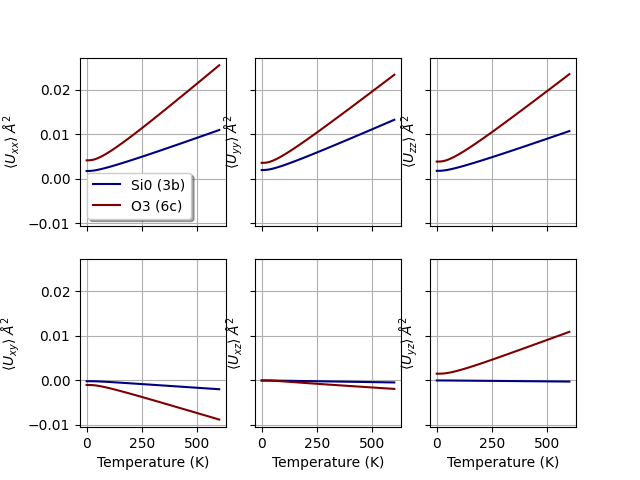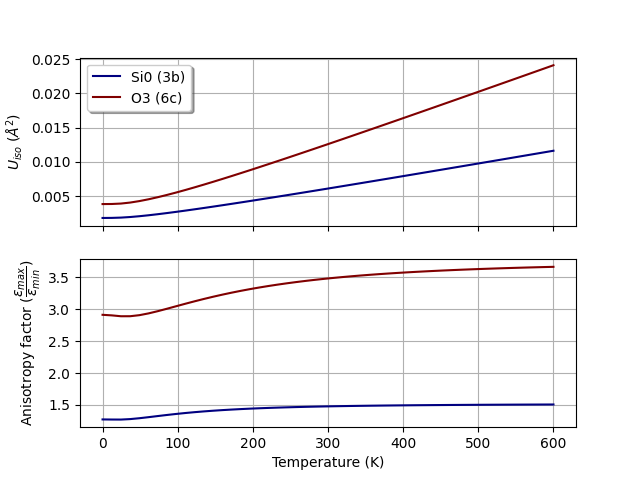# Debye-Waller and generalized phonon DOS¶

This example shows how to plot the generalized phonon DOS with the mean square displacement tensor in cartesian coords and how to calculate Debye Waller factors as a function of temperature.

See [Lee1995] for the further details about the internal implementation and [Trueblood1996] for the different conventions used by crystallographers.

•••Out:

```[33mCreating temporary file: /var/folders/nc/k69spyd12qv2tk3stk2xrxg40000gr/T/tmppv_2pfu8mp-7000_DDB[0m
```

```import os
import abipy.data as abidata

from abipy import abilab

# Open DDB file for alpha-SiO2 taken from https://materialsproject.org/materials/mp-7000/
filepath = os.path.join(abidata.dirpath, "refs", "mp-7000_DDB.bz2")
ddb = abilab.abiopen(filepath)

# Invoke anaddb to compute phonon bands and dos.
#dos_method = "gaussian"
dos_method = "tetra"
phbst_file, phdos_file = ddb.anaget_phbst_and_phdos_files(nqsmall=4, dos_method=dos_method,
ndivsm=1, mpi_procs=2)

# Extract msqd_dos
msqd_dos = phdos_file.msqd_dos
#print(msqd_dos)

#for fmt in ("cartesian", "cif", "ustar", "beta", "B"):
for fmt in ("cartesian", "cif"):
df = msqd_dos.get_dataframe(temp=300, view="all", fmt=fmt)
abilab.print_dataframe(df, title="Format: %s" % fmt)

# Plot generalized phonon DOS for each inequivalent atom in the unit cell.
msqd_dos.plot()

# Plot tensor(T) for each inequivalent atom.
msqd_dos.plot_tensor()

msqd_dos.plot_uiso()

# To save the structure and the U tensor at T=300K in CIF format, use:
#msqd_dos.write_cif_file("DW.cif", temp=300)

# To visualize the thermal ellipsoids with Vesta, use:
#msqd_dos.vesta_open(temp=300)

# Remember to close the files.
phbst_file.close()
phdos_file.close()
ddb.close()
```

Total running time of the script: ( 0 minutes 6.181 seconds)

Gallery generated by Sphinx-Gallery